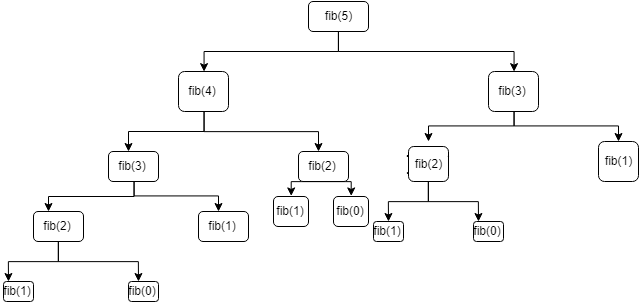Memoization and recursion with example

Posted on Nov - 2018

Understand memoization in details  with example

Memoization: It's a caching technique where the result of a function with the same argument is cached. It helps in performance optimization and is a great technique for improving the performance of recursive function with exponential time complexity.

Let's take the example of a very common interview question of solving the Fibonacci series.

Fibonacci series: It's a series of number in which each number is the sum of two preceding numbers. Ex 0, 1, 1, 2, 3, 5, 8, 13, 21 ...

Algorithms for generating the Fibonacci number at a given position n is mentioned below.

def fib
fib = {n->

if (n < 2) {
return n
}

fib(n - 1) + fib(n - 2)// last statement implicit return in groovy

}

The above program defines a closure and assigns it to variable fib which accepts n as an argument and uses recursion to get the Fibonacci number at a given index n. E.g.

fib(5) = 5

fib(6) = 8

The above recursive program goes well for the small value of but as the value of n grows the program slows down since the function call grows exponentially.

Try running fib(50)  you will realize the slow performance of the algorithm.

Fibonacci function call in pictorial form.From the above diagram, it's clear that function with the same argument is called multiples times which is unnecessary computation. We can use a technique called a memoization to cache the result the function with the same argument.

Let's write a custom memoize closure in Groovy.

def fib
fib = {n->

if (n < 2) {
return n
}

fib(n - 1) + fib(n - 2)// last statement implicit return in groovy

}
// memoize closure which takes closure as an argument

def memoize = {closure ->
def cache = [: ]

return {long args ->
if (cache[args]) {
return cache[args]
}
def result = closure.call(args)
cache[args] = result
}
}

fib = memoize(fib)
fib(100)

#13 defines a variable memoize and assigns a closure which takes a closure as a parameter and returns a closure from inside which actually does the caching using a groovy map based on the argument passed to closure.

Closures are the block of code which can be assigned to variables, passed as a parameter. Using this technique we can defer the call of closure. It can be invoked using the special call method.

#25 overrides the fib with the closures returned from the memoize closure, this is important since we use the cache to avoid expensively computation.

Run the above code you will notice a huge performance gain.

Note: Groovy has a lot of language level support for handling memoization, which can be achieved using AST as well as memoize as a method call over closure. The above code is to just explain how the memoize internally works.

Categories

 CSS Java AngularJS Groovy Grails JavaScript MongoDB React-redux SQL